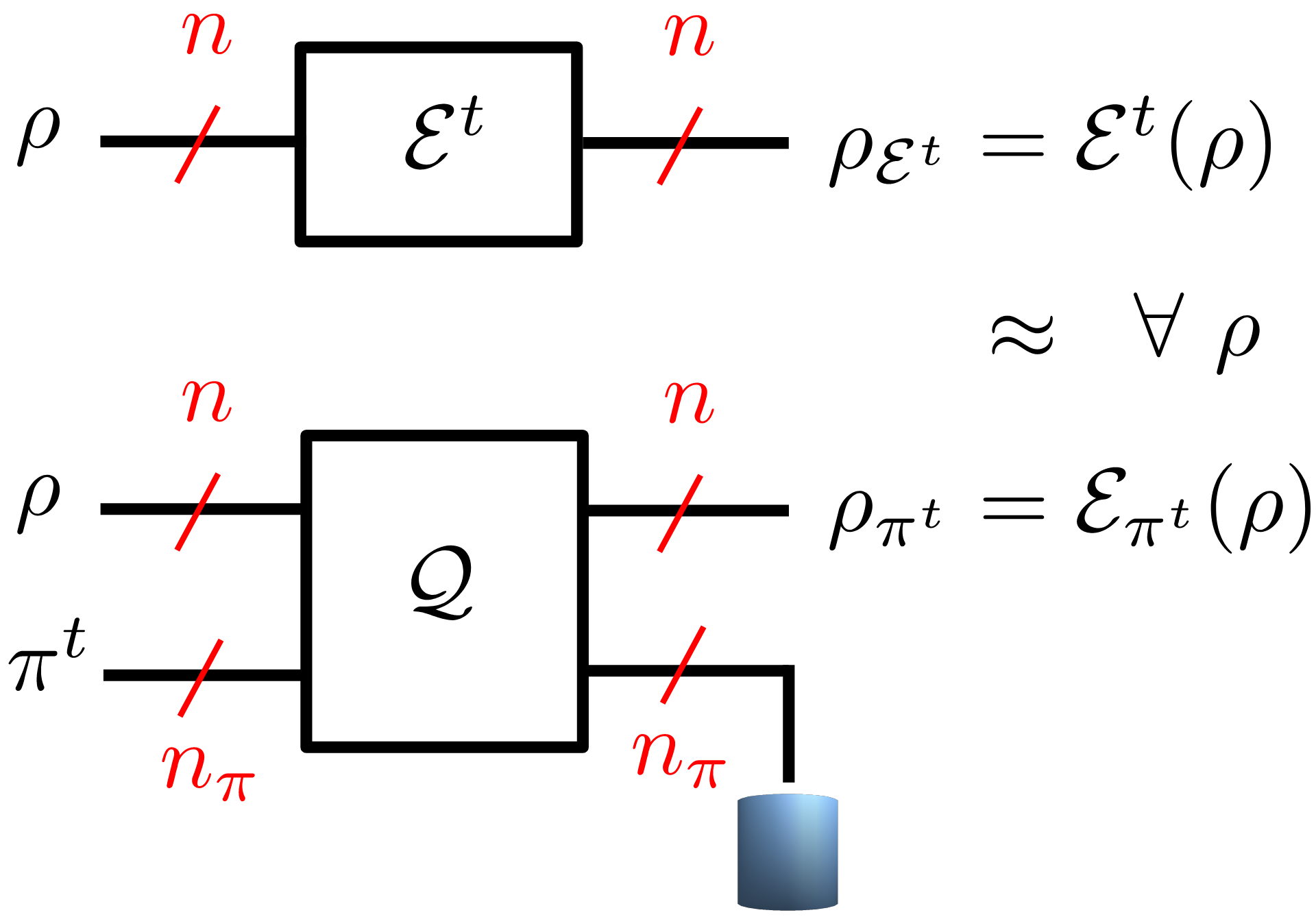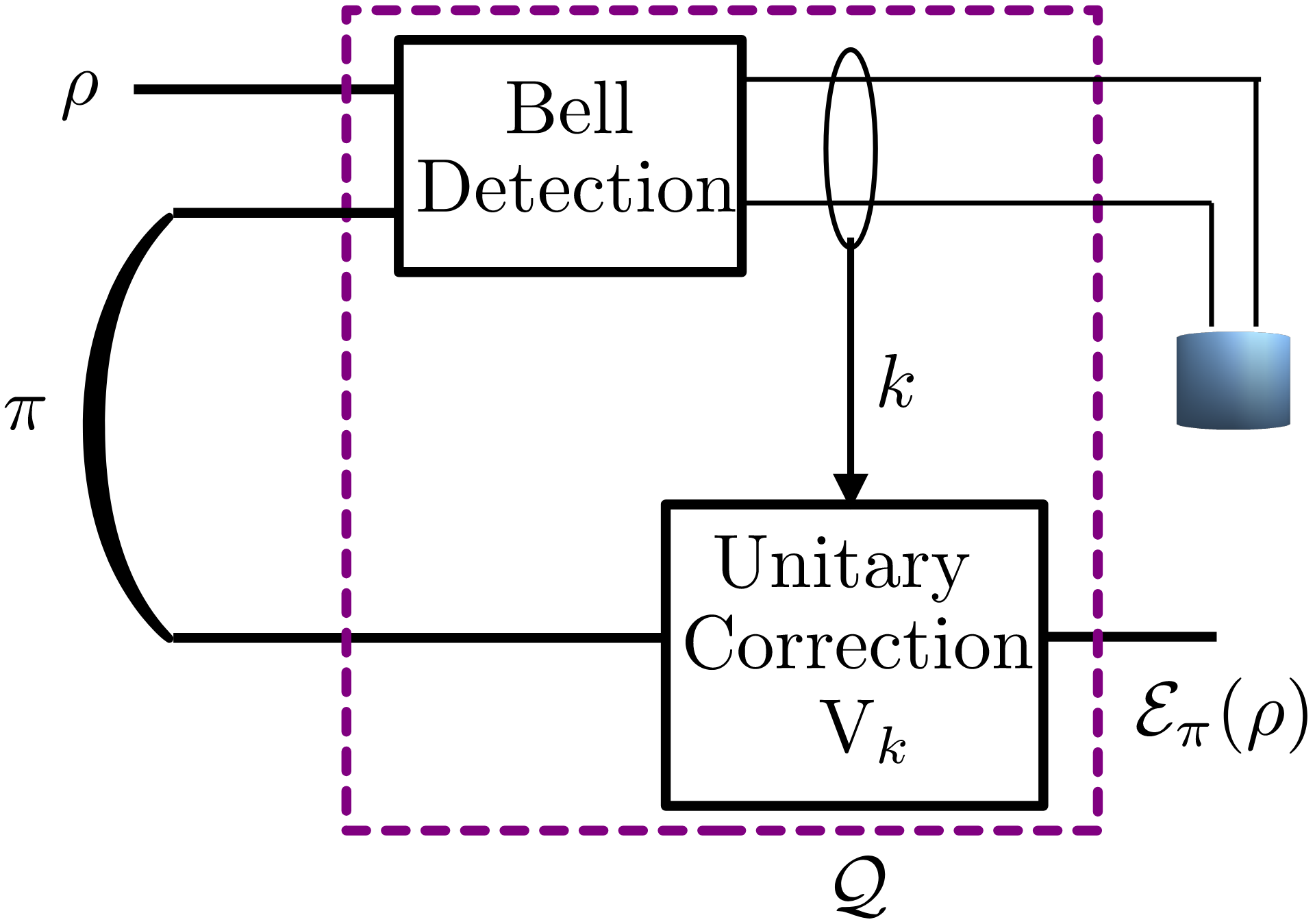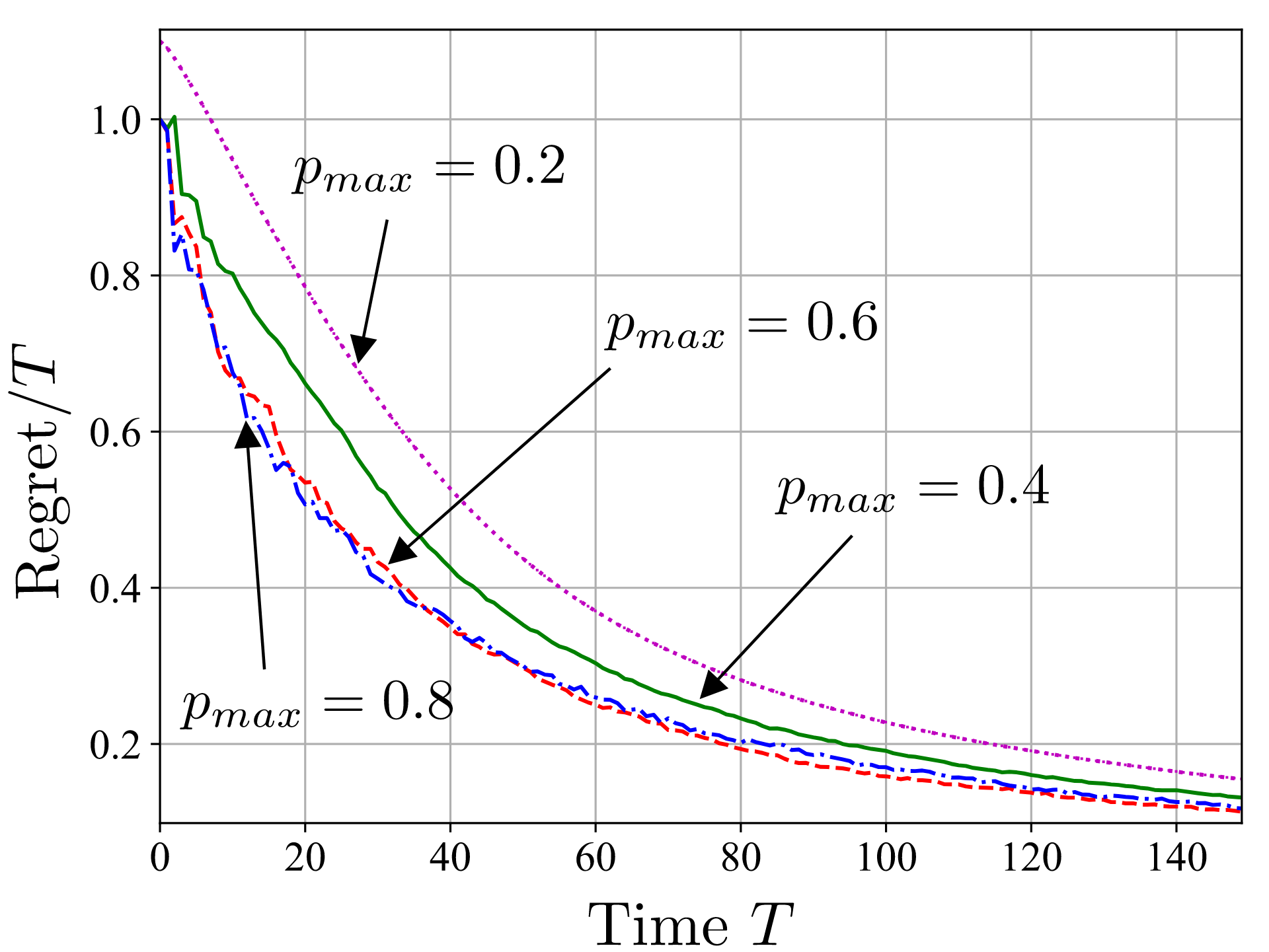A quantum computer can be programmed to carry out a given functionality in different ways, including the direct engineering of pulse sequences, the design of parametric quantum circuits via quantum machine learning (QML) , the use of adaptive measurements on cluster states, and the optimization of a program state operating on a fixed quantum processor. A fundamental result derived in  states there is no universal programmable quantum processor that operates with finite-dimensional program states. Since a quantum processor is universal if it can implement any quantum operation, this conclusion implies that the exact simulation of an arbitrary quantum channel on a single programmable quantum processor is impossible. This, in turn, highlights the importance of developing tools for the optimization of quantum programs. Reference  addressed the problem of approximately simulating a given quantum channel using a finite-dimensional program state.Fig. 1: Fig. 1. Time-varying quantum channel ε^t (top) and its simulation ε_(π^t ) via a programmable quantum processor Q controlled by the time-varying program state π^t (bottom).

In our recent work, presented at IEEE ITW 2023, we study the more challenging setting illustrated in Fig. 1, in which the channel to be simulated varies over time. We adopt a worst-case formulation in which the channel variation is arbitrary and chosen by “nature” in a possibly adversarial way. To study this setting, we propose to adopt the framework of online convex optimization , which provides tools to track the optimal solution of time-varying convex problems. We specifically develop and analyze an online mirror descent algorithm over the space of positive definite matrices, yielding a matrix exponentiated gradient descent (MEGD) . We prove that the regret of MEGD with respect to an optimized fixed program state is sublinear in time.

## ExperimentsFig. 2: Generalized teleportation processor as a programmable processor Q
operating on one input qubit (n=1) and on a two-qubit program state π
(n_π=2).

We conduct experiments by adopting the generalized teleportation processor (GTP), shown in Fig. 2, as the programmable quantum processor. GTP can simulate exactly the class of teleportation-covariant channels, modeling Pauli and erasure channels, and is operated here in an adversarial setting with time varying dephasing channels. Fig.3 plots the normalized regret as a function of time T. We observe that, MEGD is able to obtain a normalized regret that decreases sublinearly with T, hence approaching the performance of the reference program that would have been optimal in hindsight.Fig. 3: Normalized regret as a function of time T for MEGD when simulating a time-varying dephasing channel with dephasing probabilities drawn independently and uniformly at each time in the interval [0.2,p_max) (setting p_max=0.2 models a constant channel).

 O. Simeone, “An introduction to quantum machine learning for engineers”,
Foundations and Trends in Signal Processing, vol. 16, no. 1-2, pp. 1–223, 2022.

 M. A. Nielsen and I. L. Chuang, “Programmable quantum gate arrays”, Phys. Rev. Lett., vol. 79, pp. 321–324, Jul 1997.

 L. Banchi, J. Pereira, S. Lloyd, and S. Pirandola, “Convex optimization of programmable quantum computers”, npj Quantum Information, vol. 6, no. 1, pp. 1–10, 2020.

 F. Orabona, “A modern introduction to online learning”, CoRR, vol. abs/1912.13213, 2019.

 K. Tsuda, G. Ratsch, and M. K. Warmuth, “Matrix exponentiated gradient updates for on-line learning and Bregman projection”, Journal of Machine Learning Research, vol. 6, no. 34, pp. 995–1018, 2005.